# Python MySQL – Join

A connector is employed when we have to use mysql with other programming languages. The work of mysql-connector is to provide access to MySQL Driver to the required language. Thus, it generates a connection between the programming language and the MySQL Server.

#### Python-MySQL-Connector

This is a MySQL Connector that allows Python to access MySQL Driver and implement SQL queries in its programming facility. Here we will try implementing Join clause on our Database and will study the output generated.

## JOIN Clause Of SQL

Join allows you to combine two or more tables in SQL, based on related column between them. Based on this application of join there are three types of join:• INNER JOIN
gives the records that are produced by matching columns. JOIN and INNER JOIN both work the same.
Syntax:

```SELECT column1, column2...
FROM tablename
JOIN tablename ON condition;```
```SELECT column1, column2...
FROM tablename
INNER JOIN tablename ON condition;```
• LEFT JOIN
gives those records from table 1 removing exclusive contents of 2
Syntax:

```SELECT column1, column2...
FROM tablename
LEFT JOIN tablename ON condition;```
• RIGHT JOIN
gives all records from table 2 after removing exclusive records of 1.
Syntax:

```SELECT column1, column2...
FROM tablename
RIGHT JOIN tablename ON condition;```

DATABASE IN USE: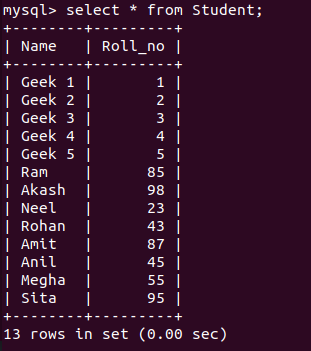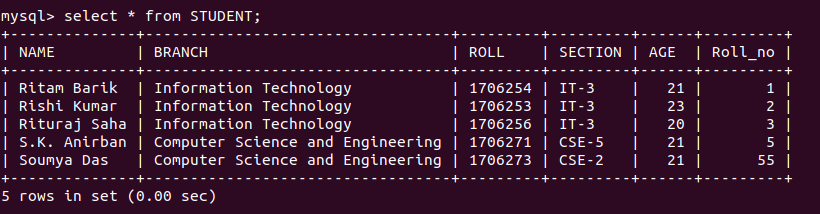PROGRAM 1: Use of inner join

 `import` `mysql.connector ` `  `  `# Conencting to the database ` `mydb ``=` `mysql.connector.connect( ` `  ``host ``=``'localhost'``, ` `  ``database ``=``'College'``, ` `  ``user ``=``'root'``, ` `) ` `  `  `cs ``=` `mydb.cursor() ` ` `  `# STUDENT and STudent are ` `# two different database ` `statement ``=``"SELECT S.NAME ``from` `Student S JOIN \ ` `Student on S.Roll_no ``=` `Student.Roll_no" ` ` `  `cs.execute(statement) ` `result_set ``=` `cs.fetchall() ` ` `  `for` `x ``in` `result_set: ` `    ``print``(x) `

OUTPUT: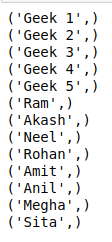PROGRAM 2: use of LEFT JOIN

 `import` `mysql.connector ` `  `  `# Conencting to the database ` `mydb ``=` `mysql.connector.connect( ` `  ``host ``=``'localhost'``, ` `  ``database ``=``'College'``, ` `  ``user ``=``'root'``, ` `) ` `  `  `cs ``=` `mydb.cursor() ` ` `  `# STUDENT and STudent are ` `# two different database ` `statement ``=``"SELECT S.Name ``from` `STUDENT S\ ` ` ``LEFT JOIN Student s ON S.Roll_no ``=` `s.Roll_no" ` ` `  `cs.execute(statement) ` `result_set ``=` `cs.fetchall() ` ` `  `for` `x ``in` `result_set: ` `    ``print``(x) `

OUTPUT: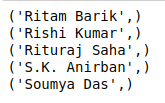PROGRAM 3 : use of RIGHT JOIN

 `import` `mysql.connector ` `  `  `# Conencting to the database ` `mydb ``=` `mysql.connector.connect( ` `  ``host ``=``'localhost'``, ` `  ``database ``=``'College'``, ` `  ``user ``=``'root'``, ` `) ` `  `  `cs ``=` `mydb.cursor() ` ` `  `# STUDENT and STudent are ` `# two different database ` `statement ``=``"SELECT S.Name ``from` `STUDENT S RIGHT \ ` `JOIN Student s ON S.Roll_no ``=` `s.Roll_no" ` ` `  `cs.execute(statement) ` `result_set ``=` `cs.fetchall() ` ` `  `for` `x ``in` `result_set: ` `    ``print``(x) `

OUTPUT: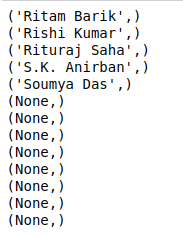My Personal Notes arrow_drop_upCheck out this Author's contributed articles.

If you like GeeksforGeeks and would like to contribute, you can also write an article using contribute.geeksforgeeks.org or mail your article to contribute@geeksforgeeks.org. See your article appearing on the GeeksforGeeks main page and help other Geeks.

Please Improve this article if you find anything incorrect by clicking on the "Improve Article" button below.

Article Tags :

Be the First to upvote.

Please write to us at contribute@geeksforgeeks.org to report any issue with the above content.